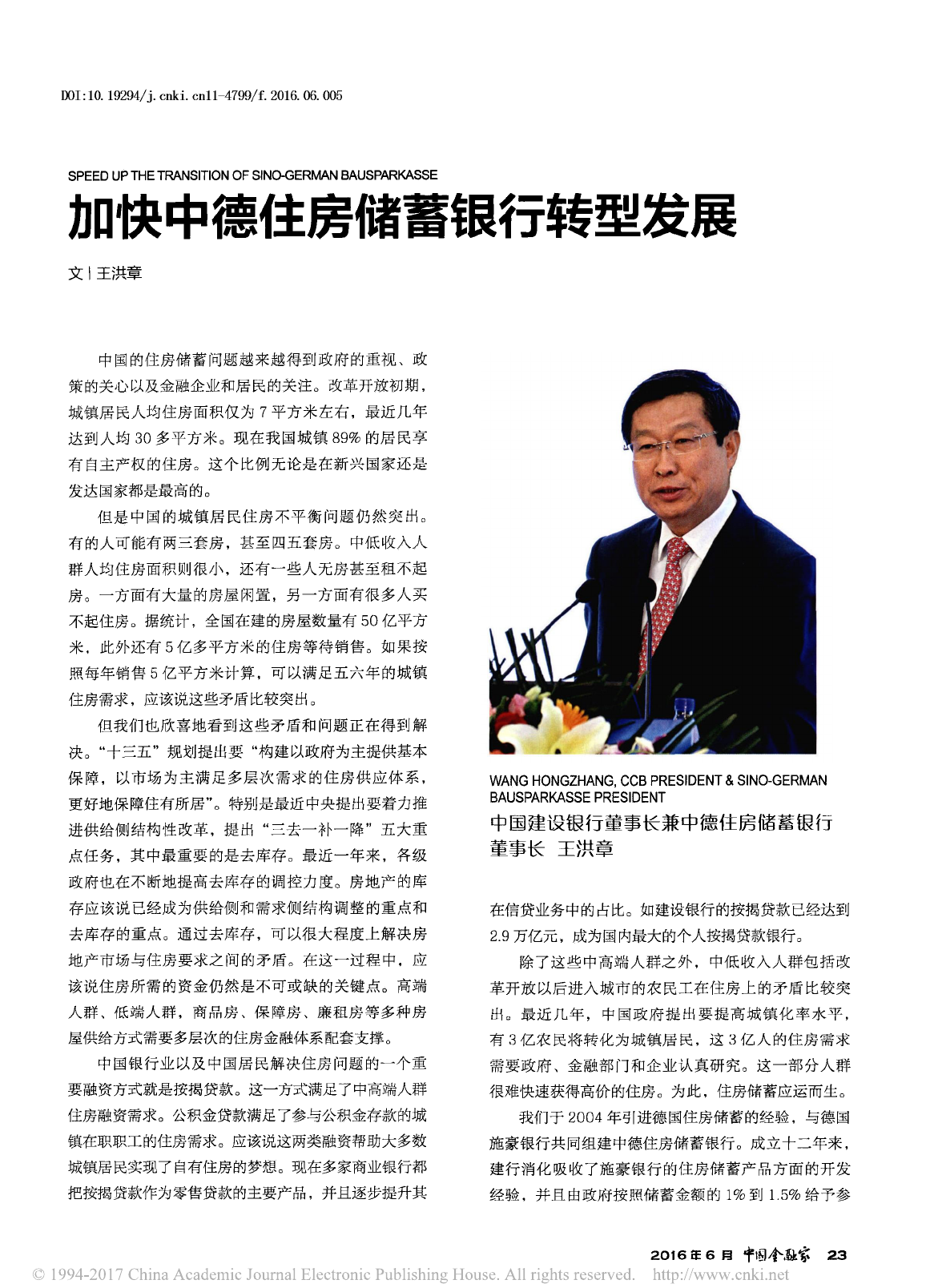加快中德住房储蓄银行转型发展S P E E D U
P T H E T R A N S I T I O N O F S I N O
-
G E R M A N B A U S P A R K A S S E
I
,
±
7
,
3 0
8 9 %
v

,
^
,

-
s a s
: . ' )
f
A
i
^
y
M m ^
_
.
n
m
e

^
,
.
W A N G H O N G Z H A N
G
, C
C
B P R E
S I D E N T & S I N O
-
G E R M A N
B M J S P A F i K A S S E P I R E S I D E M T
,
, ,
,
,
,
2 . 9

,
D
,
,
,
,
,
3

, 3 亿
,
2 0 0 4
,
,
,
,
1 % 1
.
5 %
2 0 1 6 5 6
6 )
2 3
!#%&%()+,+ ./%%(0&#1#1##2